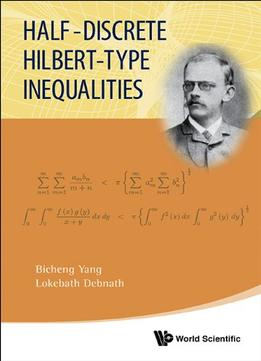# Half-Discrete Hilbert-Type Inequalities by Bicheng Yang / 2014 / English / PDF

In 1934, G. H. Hardy et al. published a book entitled "Inequalities", in which a few theorems about Hilbert-type inequalities with homogeneous kernels of degree -one were considered. Since then, the theory of Hilbert-type discrete and integral inequalities is almost built by Prof Bicheng Yang in their four published books. This monograph deals with half-discrete Hilbert-type inequalities. By means of building the theory of discrete and integral Hilbert-type inequalities, and applying the technique of Real Analysis and Summation Theory, some kinds of half-discrete Hilbert-type inequalities with the general homogeneous kernels and non- homogeneous kernels are built. The relating best possible constant factors are all obtained and proved. The equivalent forms, operator expressions and some kinds of reverses with the best constant factors are given. We also consider some multi-dimensional extensions and two kinds of multiple inequalities with parameters and variables, which are some extensions of the two-dimensional cases. As applications, a large number of examples with particular kernels are also discussed. The authors have been successful in applying Hilbert-type discrete and integral inequalities to the topic of half-discrete inequalities. The lemmas and theorems in this book provide an extensive account of these kinds of inequalities and operators. This book can help many readers make good progress in research on Hilbert-type inequalities and their applications.

views: 498